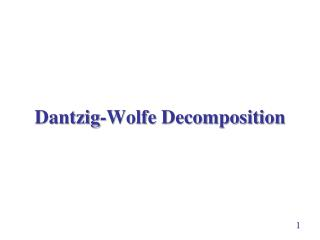Download PresentationDantzig -Wolfe Decomposition

# Dantzig -Wolfe Decomposition - PowerPoint PPT PresentationDownload Presentation## Dantzig -Wolfe Decomposition

- - - - - - - - - - - - - - - - - - - - - - - - - - - E N D - - - - - - - - - - - - - - - - - - - - - - - - - - -
##### Presentation Transcript

1. Outline • block structure of a problem • representing a point by extreme points • Revised Simplex to the extreme point representation • an example 2

2. General Problem • min • s.t. • B1x1 = b1, • B2x2 = b2, • … • BKxK = bK, • 0  xk, k = 1, 2, …, K.   • common in network-based problems • distribution of K types of products • Bkxk = bk: constraints related to the flow of the kth type of products • constraints of common resources for the K products: 3

3. General Problem • min • s.t. • B1x1 = b1, • B2x2 = b2, • … • BKxK = bK, • 0  xk, k = 1, 2, …, K.   4

4. General Problem • min • s.t. • B1x1 = b1, • B2x2 = b2, • … • BKxK = bK, • 0  xk, k = 1, 2, …, K.   Possible to avoid solving a large problem? 5

5. A Numerical Example • Problem P2: • min –3x1 – 2x2 – 2x3 – 4x4, • s.t. x1 + x2 + 2x3 + x4 10, • x1 + 2x2 8, • x2 3, • x3 + 3x4 6, • x3 4, • xi 0. 6

6. A Numerical Example • feasible region: {(x1, x2): x1 + 2x2 8, x2 3} • extreme points: • a feasible point: convex combination of the extreme points 7

7. A Numerical Example • feasible region: {(x3, x4): x3 + 3x4 6, x3 4} • extreme points: • a feasible point: convex combination of the extreme points 8

8. A Numerical Example • Problem P3: problem in terms of extreme points • min -3(812+213) – 2(313+314) – 2(422+423) – 4( 23+224), • s.t. (812+213) + (313+314) + 2(422+423) + ( 23+224)  10, • 11 + 12 + 13 + 14 = 1, • 21 + 22 + 23 + 24 = 1, • ij 0, i = 1, 2; j = 1, 2, 3, 4. a subspace Bkxk= bk is represented by a single constraint n kn = 1 9

9. A Numerical Example • Problem P3: problem in terms of extreme points • min –2412 – 1213 – 614 – 822 – (32/3)23 – 824, • s.t. 812 + 513 + 314 + 822 +8 23 +224 10, • 11 + 12 + 13 + 14 = 1, • 21 + 22 + 23 + 24 = 1, • ij 0, i = 1, 2; j = 1, 2, 3, 4. The previous representation suits DW Decomposition more. 10

10. Practical? • impractical approach • impossible to generate all the extreme points of a block Bkxk = bk • Dantzig-Wolfe Decomposition: check all extremely points without explicitly generating them 11

11. General Problem • K blocks,Bkxk = bk, k = 1, …, K • Nk extreme points in the kth block dual variable 0  dual variable 1  dual variable 2  … dual variable K  12

12. Reduced Cost for a Non-Basic Variable kn • reduced cost of kn 13

13. Most Negative Reduced Cost where Check each extreme point for each block, which is equivalent to solving a linear program. Result: Solving K+1 small linear programs. 14

14. General Approach • 1 Form the master program (MP) by the representation • 2 Get a feasible solution of the MP; find the corresponding  • 3 Solve the subproblems to check the reduced costs of kn • 3.1 stop if the MP is optimal; • 3.2 else carry a standard revised simplex iteration (i.e., identifying the entering and leaving variables, stopping for an unbounded problem , and determining B-1 otherwise) • 3.3 go back to 2 if the problem is not unbounded 15

15. An Example of the Dantzig-Wolfe Approach • min –3y1 – 2y2 – 2y3 – 4y4, • s.t. y1 + y2 + 2y3 + y4 10, • y1 + 2y2 8, • y2 3, • y3 + 3y4 6, • y3 4, • yi 0. 16

16. An Example of the Dantzig-Wolfe Approach • problem in general form with 17

17. An Example of the Dantzig-Wolfe Approach • let be the extreme points of B1x1 = b1 • any point in B1x1 = b1: • similarly, any point in B2x2 = b2: 18

18. An Example of the Dantzig-Wolfe Approach • in terms of the extreme points 19

19. An Example of the Dantzig-Wolfe Approach • to solve the problem, introduce the slack variable x5 and artificial variables x6 and x7 20

20. An Example of the Dantzig-Wolfe Approach • initial basic variable xB = (x5, x6, x7)T, B = I, (cB)T = (0, M, M), b = (10, 1, 1)T •  = (cB)TB = (0, M, M) • for the kth subproblem, the reduced costs: 21